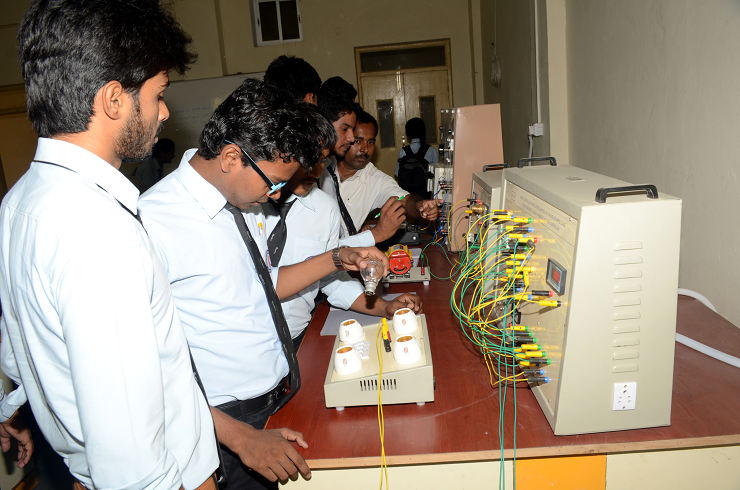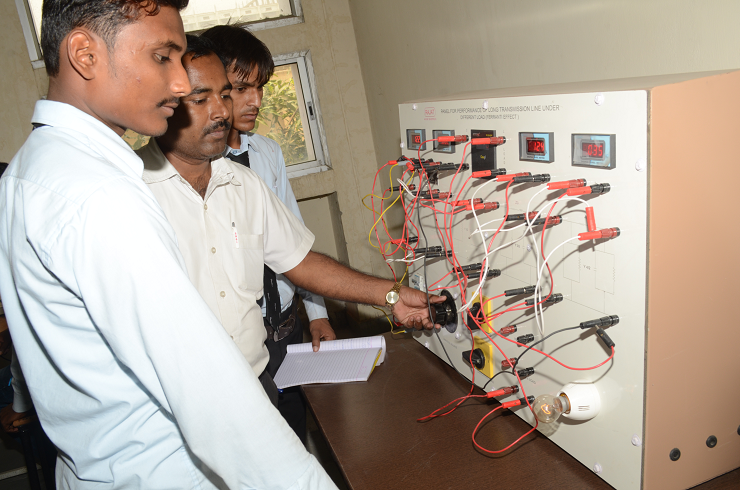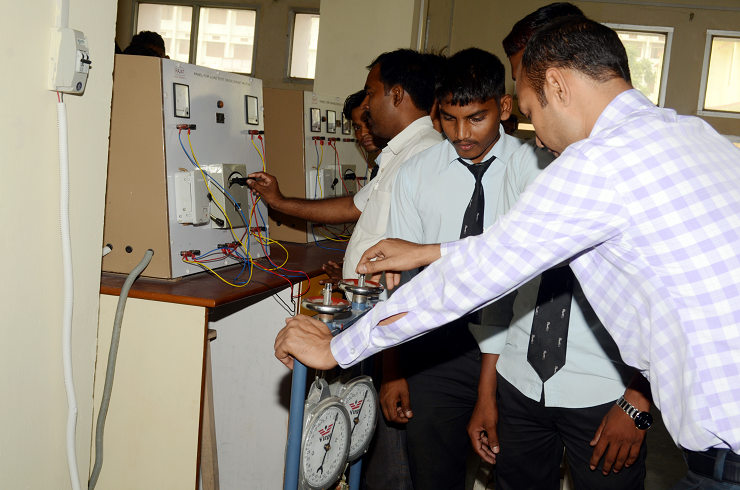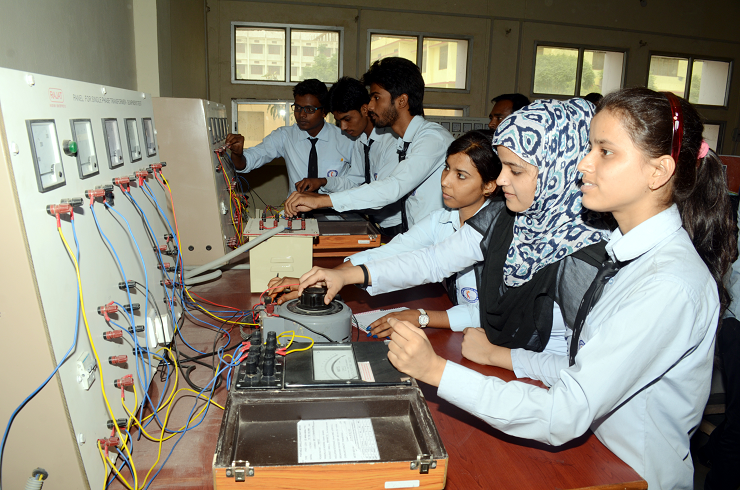## Labs##### Power Electronics LAB

1. Demonstrate the characteristics and triggering of IGBT, MOSFET, Power transistor and SCR.
2. Analyze the performance of single phase fully controlled bridge rectifiers under different loading conditions.
3. Develop simulation models of power electronic circuits

##### Power System LAB

1. Use programming tools /Software: Scilab, MATLAB or any C, C++ -Compiler and formulate a program/simulation model for calculation of various parameters related to transmission line.##### Basic Electrical LAB

1. Conduct experiments illustrating the application of KVL/KCL and network theorems to DC electrical circuits.
2. Demonstrate the behavior of AC circuits connected to single phase AC supply and measure power in single phase as well as three phase electrical circuits.
3. Calculate efficiency of a single phase transformer and DC machine.
4. Identify the type of DC and AC machines based on their construction.

##### Electrical Machines LAB

1. Analyze and conduct basic tests on DC Machines and single-phase Transformer
2. Determine the magnetization, Load and speed-torque characteristics of DC Machines.
3. Demonstrate procedures and analysis techniques to perform electromagnetic and electromechanical tests on electrical machines.##### Network System LAB

1. Apply the knowledge of basic circuital law, nodal and mesh analysis for given circuit.
2. Analysis of the AC and DC circuits using simulation techniques.
3. Analysis of transient response of AC circuits.
4. Evaluation and analysis of two-port network parameters

##### Electrical Measurement LAB

1. Understand the importance of calibration of measuring instruments.
2. Demonstrate the construction and working of different AC and DC bridges, along with their applications
3. Ability to measure electrical engineering parameters like voltage, current, power & phase difference in industry as well as in power generation, transmission and distribution sectors.